• python绘制直方图
千次阅读
2022-01-14 22:18:50

一、直方图绘制参数详解

1、plt.hist(x, bins=10, range=None, normed=False, weights=None, cumulative=False, bottom=None, histtype='bar', align='mid', orientation='vertical', rwidth=None, log=False, color=None, label=None, stacked=False)

• x：指定要绘制直方图的数据。
• bins：指定直方图条形的个数。
• range：指定直方图数据的上下界，默认包含绘图数据的最大值和最小值。
• normed：是否将直方图的频数转换成频率。
• weights：该参数可为每一个数据点设置权重。
• cumulative：是否需要计算累计频数或频率。
• bottom：可以为直方图的每个条形添加基准线，默认为0。
• histtype：指定直方图的类型，默认为bar，除此之外，还有barstacked、step和stepfilled
• align：设置条形边界值的对齐方式，默认为mid，另外还有left和right
• orientation：设置直方图的摆放方向，默认为垂直方向
• rwidth：设置直方图条形的宽度
• log：是否需要对绘图数据进行log变换
• color：设置直方图的填充色
• edgecolor：设置直方图边框色
• label：设置直方图的标签，可通过legend展示其图例
• stacked：当有多个数据时，是否需要将直方图呈堆叠摆放，默认水平摆放
# 直方图|hist()函数
import matplotlib.pyplot as plt
import pandas as pd

plt.hist(df["a36"]) # 用名称为“a36”的这列数据
plt.show()  # 绘制
更多相关内容
• 今天小编就为大家分享一篇python绘制直方图和密度图的实例，具有很好的参考价值，希望对大家有所帮助。一起跟随小编过来看看吧
• 本文主要总结如何绘制直方图，以及常用的使用场景。 什么是直方图：一个随机变量在各个取值区间有个概率分布，将其绘制出来：x轴为等间隔的取值区间(bins)，y轴为该区间的频数(可归一化)，即直方图。 接口 Signature...
• 主要介绍了详解用Python直方图绘制拟合曲线的两种方法，文中通过示例代码介绍的非常详细，对大家的学习或者工作具有一定的参考学习价值，需要的朋友们下面随着小编来一起学习学习吧

本文主要介绍两个内容：

如何使用记事本生成包含某一数据集的CSV文件；

如何使用Python绘制给定数据集的直方图和正态分布曲线。

1. 使用记事本创建CSV文件

① 新建一个文本文件，打开后输入数据，格式如下：

Mike,20,shanghai

这里需要注意的是：关键字之间使用英文逗号隔开；第一行为引用字段，第二行为对应值。

② 将文本文件另存为CSV文件，如下：

依次选择【文件】→【另存为】→【文件名：xxx.csv】→【保存类型：所有文件】→【编码：utf-8】→【保存】，图示如下：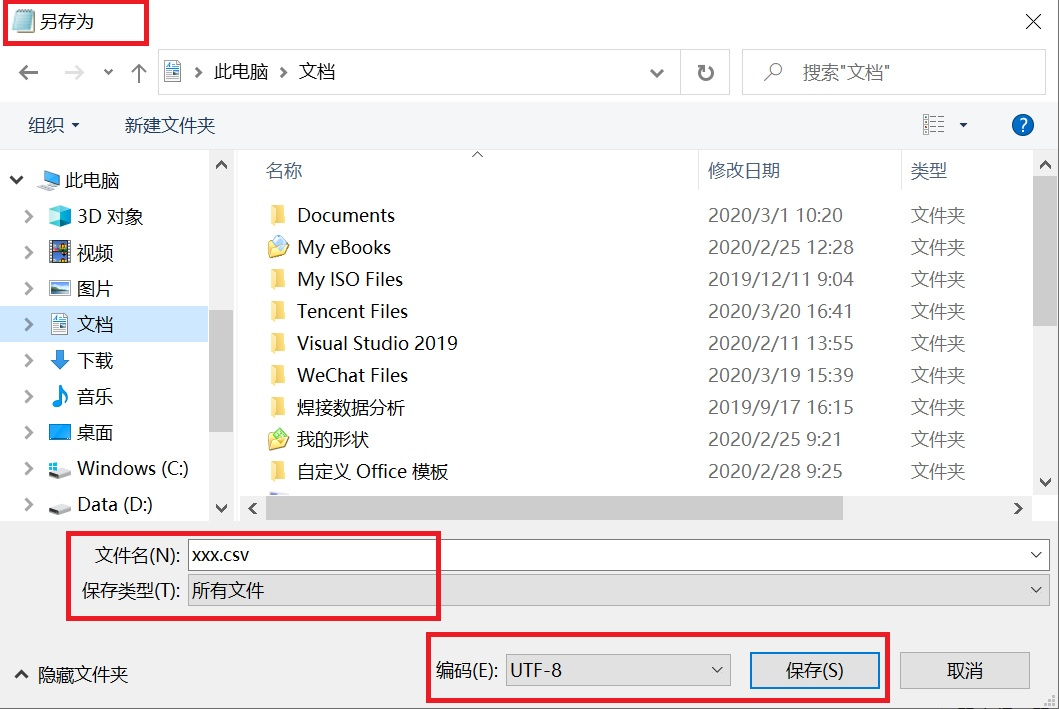③ CSV文件中的数据为100个表示长度的数值，如下：

2539 2536 2534 2542 2545 2538 2539 2542 2547 2535

2541 2543 2544 2548 2545 2543 2546 2540 2551 2545

2540 2539 2541 2536 2538 2531 2556 2543 2540 2538

2537 2544 2533 2546 2540 2549 2534 2542 2550 2537

2535 2532 2545 2540 2527 2543 2554 2539 2545 2543

2540 2543 2544 2541 2553 2537 2538 2524 2544 2540

2536 2542 2539 2546 2538 2535 2531 2534 2540 2536

2541 2532 2538 2542 2540 2533 2537 2541 2549 2535

2547 2534 2530 2539 2536 2546 2529 2540 2537 2533

2540 2535 2541 2537 2547 2539 2542 2547 2538 2539

2. 绘制数据集的直方图和正态分布曲线

1 #2 #本文以某一批产品的长度为数据集

3 #在此数据集的基础上绘制直方图和正态分布曲线

4 #5

6 import pandas as pd #pandas是一个强大的分析结构化数据的工具集

7 import numpy as np #numpy是Python中科学计算的核心库

8 import matplotlib.pyplot as plt #matplotlib数据可视化神器

9

10 #正态分布的概率密度函数

11 #x 数据集中的某一具体测量值

12 #mu 数据集的平均值，反映测量值分布的集中趋势

13 #sigma 数据集的标准差，反映测量值分布的分散程度

14 defnormfun(x, mu, sigma):15 pdf = np.exp(-((x - mu) ** 2) / (2 * sigma ** 2)) / (sigma * np.sqrt(2 *np.pi))16 returnpdf17

18 if __name__ == '__main__':19

21 length = data['length'] #获得长度数据集

22 mean = length.mean() #获得数据集的平均值

23 std = length.std() #获得数据集的标准差

24

25 #设定X轴：前两个数字是X轴的起止范围，第三个数字表示步长

26 #步长设定得越小，画出来的正态分布曲线越平滑

27 x = np.arange(2524, 2556, 0.1)28 #设定Y轴，载入刚才定义的正态分布函数

29 y =normfun(x, mean, std)30 #绘制数据集的正态分布曲线

31 plt.plot(x, y)32

33 #绘制数据集的直方图

34 plt.hist(length, bins=12, rwidth=0.9, density=True)35 plt.title('Length distribution')36 plt.xlabel('Length')37 plt.ylabel('Probability')38

39 #输出正态分布曲线和直方图

40 plt.show()

程序执行结果如下：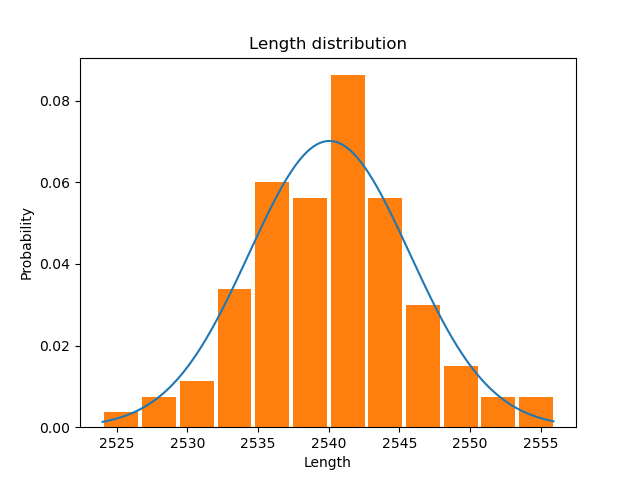展开全文本文主要介绍两个内容：

如何使用记事本生成包含某一数据集的CSV文件；

如何使用Python绘制给定数据集的直方图和正态分布曲线。

1. 使用记事本创建CSV文件

① 新建一个文本文件，打开后输入数据，格式如下：

Mike,20,shanghai

这里需要注意的是：关键字之间使用英文逗号隔开；第一行为引用字段，第二行为对应值。

② 将文本文件另存为CSV文件，如下：

依次选择【文件】→【另存为】→【文件名：xxx.csv】→【保存类型：所有文件】→【编码：utf-8】→【保存】，图示如下：③ CSV文件中的数据为100个表示长度的数值，如下：

2539 2536 2534 2542 2545 2538 2539 2542 2547 2535

2541 2543 2544 2548 2545 2543 2546 2540 2551 2545

2540 2539 2541 2536 2538 2531 2556 2543 2540 2538

2537 2544 2533 2546 2540 2549 2534 2542 2550 2537

2535 2532 2545 2540 2527 2543 2554 2539 2545 2543

2540 2543 2544 2541 2553 2537 2538 2524 2544 2540

2536 2542 2539 2546 2538 2535 2531 2534 2540 2536

2541 2532 2538 2542 2540 2533 2537 2541 2549 2535

2547 2534 2530 2539 2536 2546 2529 2540 2537 2533

2540 2535 2541 2537 2547 2539 2542 2547 2538 2539

2. 绘制数据集的直方图和正态分布曲线

1 #2 #本文以某一批产品的长度为数据集

3 #在此数据集的基础上绘制直方图和正态分布曲线

4 #5

6 import pandas as pd #pandas是一个强大的分析结构化数据的工具集

7 import numpy as np #numpy是Python中科学计算的核心库

8 import matplotlib.pyplot as plt #matplotlib数据可视化神器

9

10 #正态分布的概率密度函数

11 #x 数据集中的某一具体测量值

12 #mu 数据集的平均值，反映测量值分布的集中趋势

13 #sigma 数据集的标准差，反映测量值分布的分散程度

14 defnormfun(x, mu, sigma):15 pdf = np.exp(-((x - mu) ** 2) / (2 * sigma ** 2)) / (sigma * np.sqrt(2 *np.pi))16 returnpdf17

18 if __name__ == '__main__':19

21 length = data['length'] #获得长度数据集

22 mean = length.mean() #获得数据集的平均值

23 std = length.std() #获得数据集的标准差

24

25 #设定X轴：前两个数字是X轴的起止范围，第三个数字表示步长

26 #步长设定得越小，画出来的正态分布曲线越平滑

27 x = np.arange(2524, 2556, 0.1)28 #设定Y轴，载入刚才定义的正态分布函数

29 y =normfun(x, mean, std)30 #绘制数据集的正态分布曲线

31 plt.plot(x, y)32

33 #绘制数据集的直方图

34 plt.hist(length, bins=12, rwidth=0.9, density=True)35 plt.title('Length distribution')36 plt.xlabel('Length')37 plt.ylabel('Probability')38

39 #输出正态分布曲线和直方图

40 plt.show()

程序执行结果如下：展开全文• 本文主要介绍一下 Python 怎么绘制直方图，涉及到直方图分组绘制，标签，图例，坐标轴的设置。 **内容提要:** 1. Matplotlib 简介 Pyplot 模块 2. plt.figure 创建画板 3. plt.bar 绘制直方图 单组直方图的例子 ...

# 引言

本文主要介绍一下 Python 怎么绘制直方图，涉及到直方图分组绘制，标签，图例，坐标轴的设置。

内容提要:

1. Matplotlib 简介
Pyplot 模块
2. plt.figure 创建画板
3. plt.bar 绘制直方图
单组直方图的例子
分组直方图的例子
4. plt.text 设置数值标签
5. plt.legend() 设置图例
6. plt.gca() 坐标轴的设置
7. 一个完整的直方图例子

# Matplotlib 简介

Matplotlib 是 Python 的绘图库，它能让使用者很轻松地将数据图形化，并且提供多样化的输出格式。 Matplotlib 是一个非常强大的 Python 画图工具，我们可以使用该工具将很多数据通过图表的形式更直观的呈现出来。Matplotlib 可以绘制线图、散点图、等高线图、条形图、柱状图、3D 图形、甚至是图形动画等等。

要使用 Matplotlib，我们需要先安装再导入
pip install matplotlib
import matplotlib

## Pyplot

Pyplot 是 Matplotlib 的子库，提供了和 MATLAB 类似的绘图 API。Pyplot 是常用的绘图模块，能很方便让用户绘制 2D 图表。Pyplot 包含一系列绘图函数的相关函数，每个函数会对当前的图像进行一些修改，例如：给图像加上标记，生新的图像，在图像中产生新的绘图区域等等。更多信息，请考官网

使用的时候，我们可以使用 import 导入 pyplot 库，并设置一个别名 plt：
import matplotlib.pyplot as plt

# plt.figure 函数

创建一个画板

matplotlib.pyplot.figure(num=None, figsize=None, dpi=None, facecolor=None, edgecolor=None, frameon=True, FigureClass=<class ‘matplotlib.figure.Figure’>, clear=False, **kwargs)

参数表述
num指定创建的 figure 名称，默认按创建的顺序构建数字，文本类型；
figsize以英寸为单位的宽高(1英寸等于2.54 厘米)，用元组表示 figsize=(15,3)；默认创建一个大小为 432x288 大小的画板(单位是像素)
dpi指定绘图对象的分辨率，即每英寸多少个像素，缺省值为 72
facecolor背景颜色
edgecolor边框颜色
frameon默认值True为绘制边框，如果为False则不绘制边框；
FigureClass可以选择使用自定义图形实例；
clear重建figure实例；
**kwargs允许将自定义的图类绑定到pylab接口中，额外的kwargs将被传递给figure init函数。

下面通过例子来说明一下 figsize 和 dpi 怎么影响画板尺寸的，为了更形象，我们在画本里添加一个线性图。

import matplotlib.pyplot as plt

def plot(fs, dpi_set):
plt.figure(figsize=fs, dpi=dpi_set)
plt.title("size:{}, dpi:{}".format(fs, dpi_set))
plt.plot([0, 1, 2, 3], [3, 4, 2, 5])
plt.savefig(str(fs) + "-" + str(dpi_set) + ".png")

if __name__ == "__main__":
figsize = (2, 2)
for i in range(1, 4):
plot(figsize, i*72)

for i in [2, 4, 6]:
plot((i, i), 72)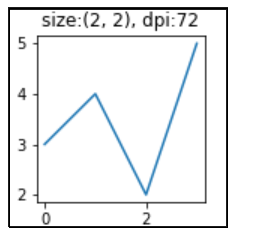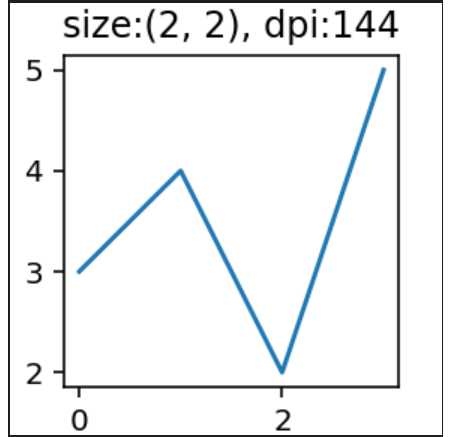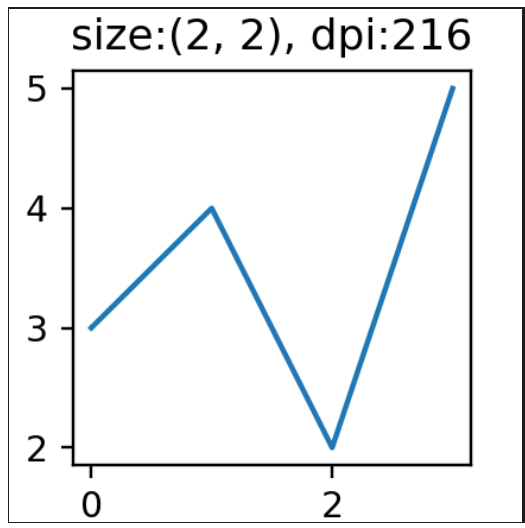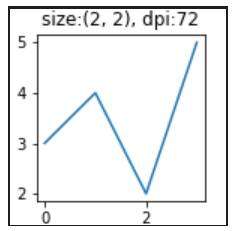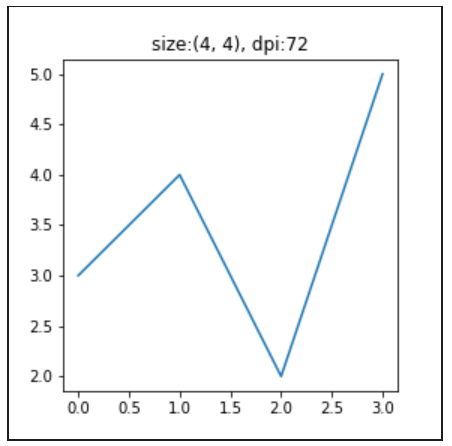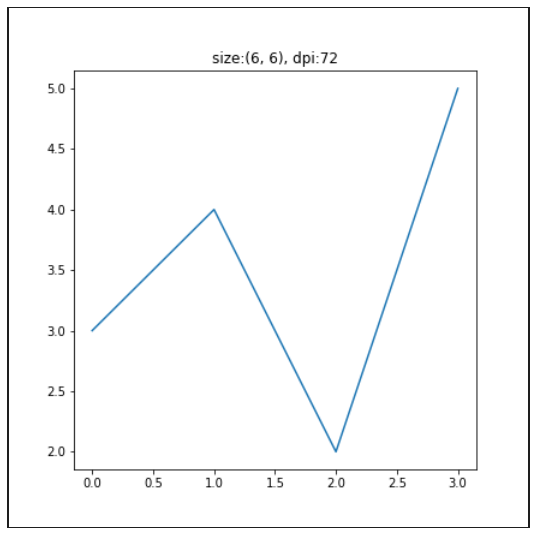# plt.bar 函数

绘制直方图

matplotlib.pyplot.bar(x, height, width=0.8, bottom=None, *, align=‘center’, data=None, **kwargs)

参数描述
x为一个标量序列，确定 x 轴刻度数目
height确定 y 轴的刻度, 可以是一个标量序列或一个标量
width单个直方图的宽度，可以是一个标量序列或一个标量，默认 0.8
bottom设置 y 边界坐标轴起点，可以是一个标量序列或一个标量
color设置直方图颜色（只给出一个值表示全部使用该颜色，若赋值颜色列表则会逐一染色，若给出颜色列表数目少于直方图数目则会循环利用
edgecolor直方图边框颜色

## 单组直方图的例子：

   import matplotlib.pyplot as plt

x=[1,2,3,4,5]
y=[5,7,4,3,1]
color=['red','black','peru','orchid','deepskyblue']
x_label=['One','Two','Three','Four','Five']
plt.xticks(x, x_label)
plt.bar(x, y,color=color)

plt.show()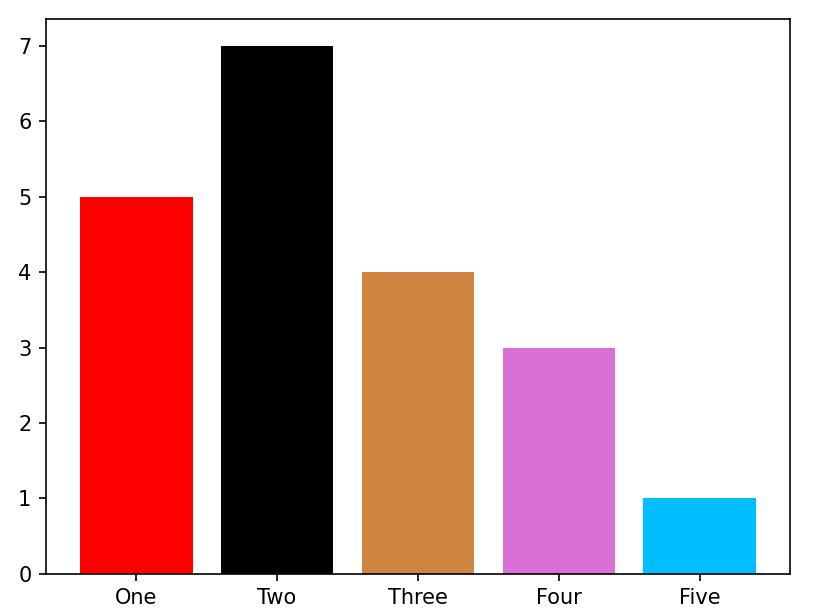改变柱形图的宽度

plt.bar(x, y,width=[0.1,0.2,0.3,0.4,0.5],color=color)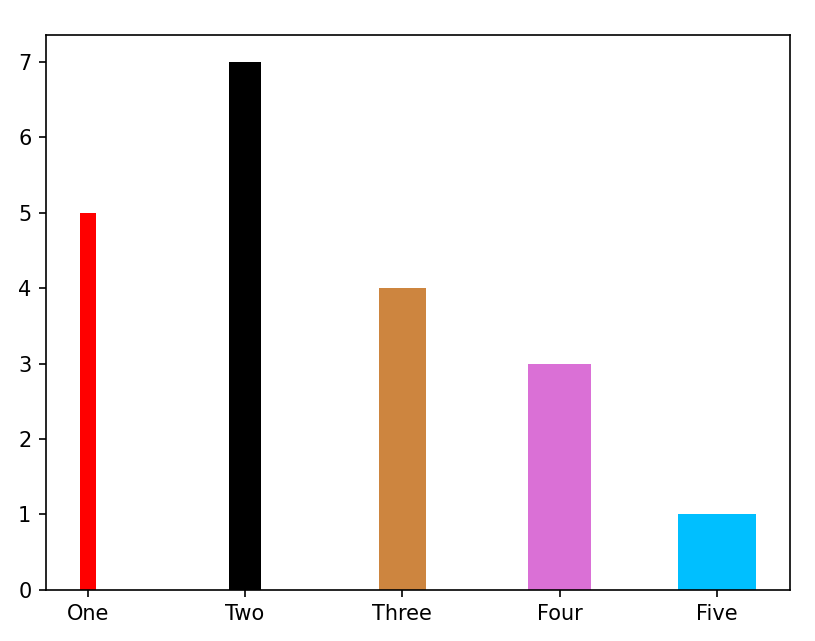将颜色设置成只有两个：会循环使用

color=['red','black']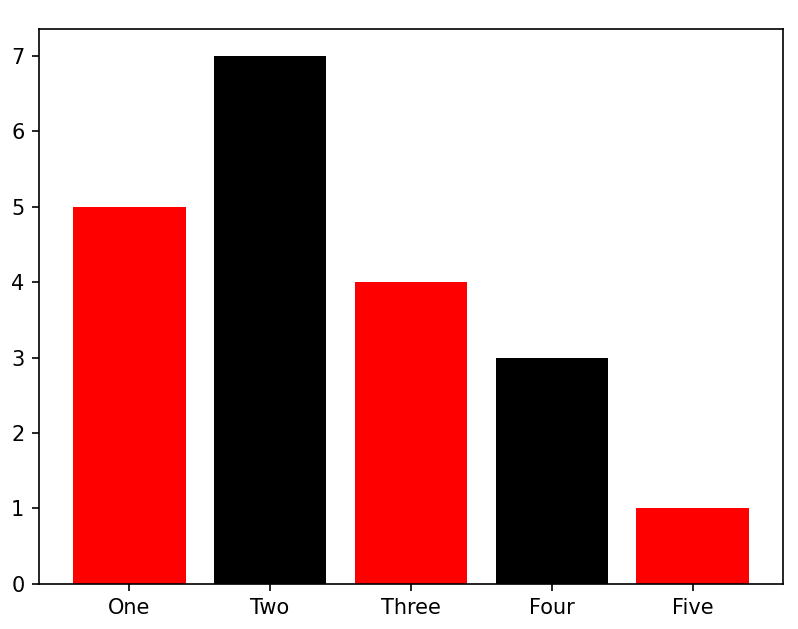## 分组直方图

有时我们进行不同类数据对比，例如统计不同时间 regression test cases 和 smoke test cases 运行情况情况。

    import matplotlib.pyplot as plt
import numpy as np

date = ['2022/2/1', '2022/2/2', '2022/2/3', '2022/2/4', '2022/2/5']
regression_test_cases_pass_total = [10, 20, 30, 40, 50]
smoke_test_cases_pass_total = [11, 12, 13, 14, 15]

x = np.arange(len(date))  # the label locations
width = 0.35  # the width of the bars

plt.bar(x - width/2, regression_test_cases_pass_total, width, label='Regression passed')
plt.bar(x + width/2, smoke_test_cases_pass_total, width, label='Smoke passed')

# Add some text for labels, title and custom x-axis tick labels, etc.
plt.ylabel('Count')
plt.xlabel('Date')
plt.title('Test Result Trend')
plt.xticks(x,date)
plt.legend()
plt.show()


这里的亮点在于：调整每个刻度上的两个直方图，使其分列刻度两边
这里通过左移和右移柱状图，达到互相“礼让”的目的
x - width/2 在 x 处，左移直方图宽度一半的距离
x + width/2 在 x 处，右移直方图宽度一半的距离

  plt.bar(x - width/2, regression_test_cases_pass_total, width, label='Regression passed')
plt.bar(x + width/2, smoke_test_cases_pass_total, width, label='Smoke passed')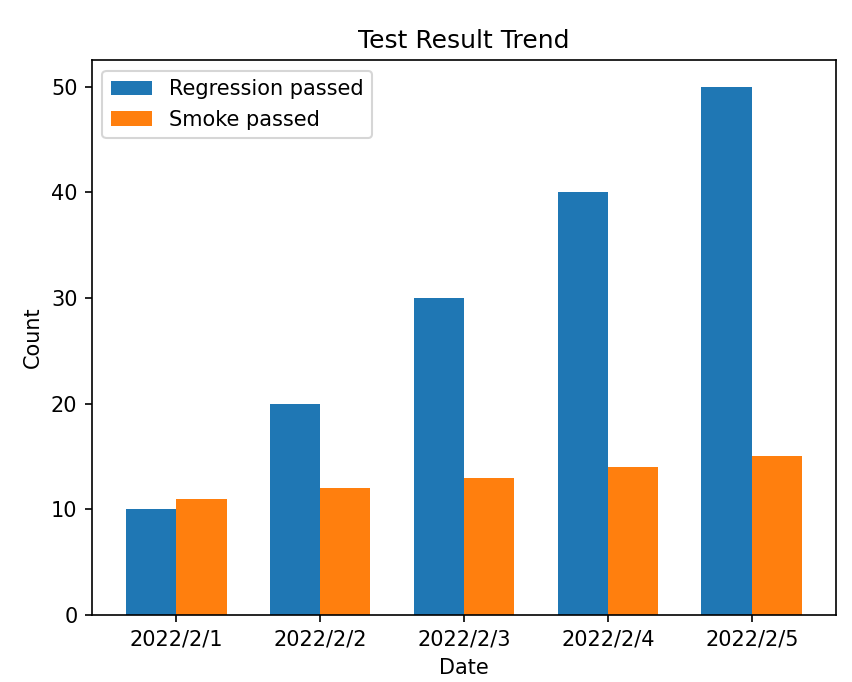如果不调整柱状图的位置，会造成同一刻度的柱状图重叠
后赋值的（Smoke 的）会遮盖先赋值绘图的 Regression 的

plt.bar(x, regression_test_cases_pass_total, width, label='Regression passed')
plt.bar(x, smoke_test_cases_pass_total, width, label='Smoke passed')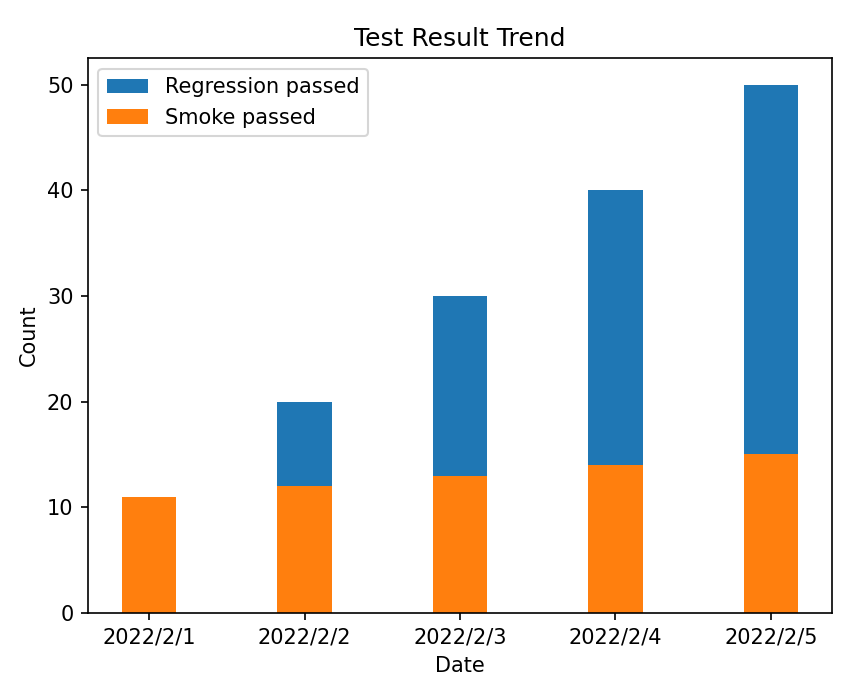通过设置 bottom 来设置 Y 轴的位置，默认的 Y 轴位置是从 0 开始，如下面通过 bottom=regression_test_cases_pass_total 将 smoke 的数据绘制在 regression 数据的上方，这里可不是重叠，只是将 smoke Y 轴的位置抬高了。

plt.bar(x, regression_test_cases_pass_total, width, label='Regression passed')
plt.bar(x, smoke_test_cases_pass_total, width, bottom=regression_test_cases_pass_total,label='Smoke passed')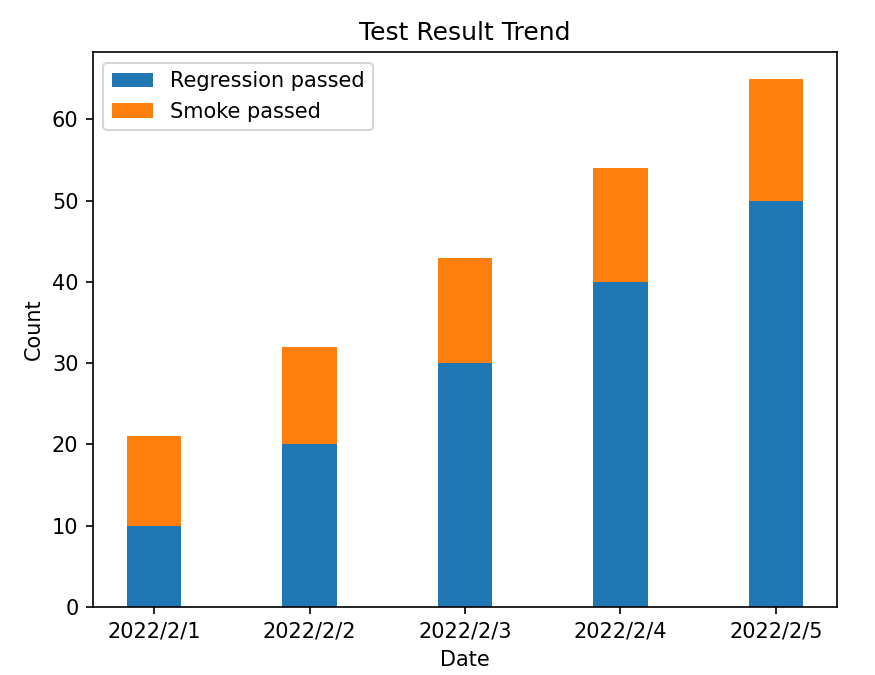# plt.text 设置数值标签

给直方图标上数值标签，使得数据一目了然。

matplotlib.pyplot.text(x, y, s, fontdict=None, withdash=, **kwargs)

参数描述
x,y表示标签添加的位置，默认是根据坐标轴的数据来度量的，是绝对值，也就是说图中点所在位置的对应的值
s显示内容
fontdict一个定义s格式的dict
fontsize字体大小
colorstr or tuple, 设置字体颜色 ,单个字符候选项{‘b’, ‘g’, ‘r’, ‘c’, ‘m’, ‘y’, ‘k’, ‘w’}，也可以’black’,'red’等，tuple时用[0,1]之间的浮点型数据，RGB或者RGBA, 如: (0.1, 0.2, 0.5)、(0.1, 0.2, 0.5, 0.3)等
backgroundcolor字体背景颜色
horizontalalignment(ha)设置垂直对齐方式，可选参数：left,right,center
verticalalignment(va)设置水平对齐方式 ，可选参数 ： ‘center’ , ‘top’ , ‘bottom’ ,‘baseline’
rotation(旋转角度)可选参数为:vertical,horizontal 也可以为数字
alpha透明度，参数值0至1之间

看一下效果图：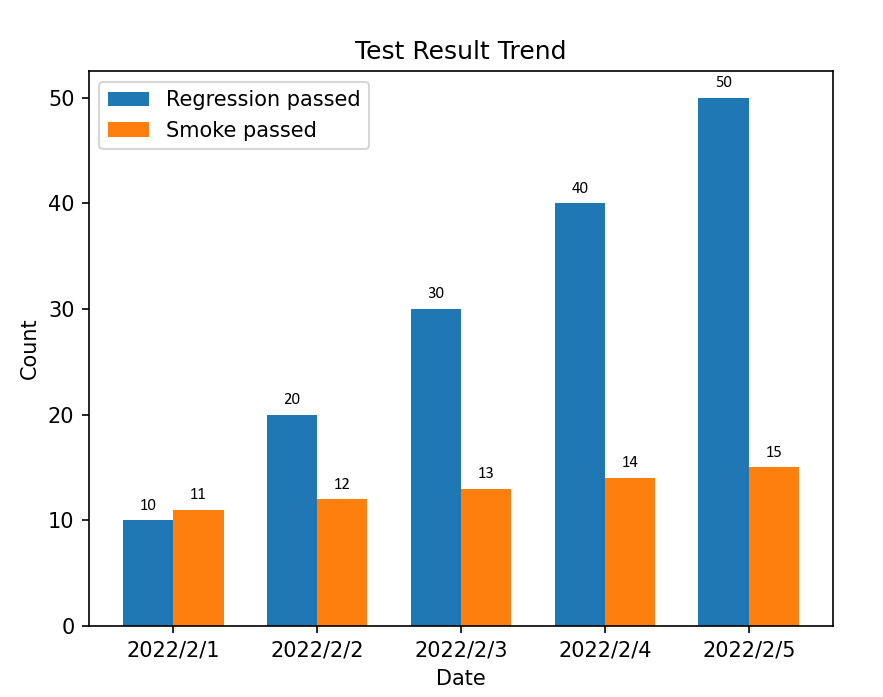通过下面语句便可实现，为了标签显示更美观，可以在 Y 轴的高度上适当调整一下，离柱状图高一点距离

    for i in x:
plt.text(i - width/2, regression_test_cases_pass_total[i] + 1,regression_test_cases_pass_total[i], ha='center', fontsize=8,family='Calibri')
plt.text(i + width/2, smoke_test_cases_pass_total[i] + 1,smoke_test_cases_pass_total[i], ha='center', fontsize=8,family='Calibri')


完整代码：

    import matplotlib.pyplot as plt
import numpy as np

date = ['2022/2/1', '2022/2/2', '2022/2/3', '2022/2/4', '2022/2/5']
regression_test_cases_pass_total = [10, 20, 30, 40, 50]
smoke_test_cases_pass_total = [11, 12, 13, 14, 15]

x = np.arange(len(date))  # the label locations
width = 0.35  # the width of the bars

plt.bar(x - width/2, regression_test_cases_pass_total, width, label='Regression passed')
plt.bar(x + width/2, smoke_test_cases_pass_total, width, label='Smoke passed')

# Add some text for labels, title and custom x-axis tick labels, etc.
plt.ylabel('Count')
plt.xlabel('Date')
plt.title('Test Result Trend')
plt.xticks(x,date)

for i in x:
plt.text(i - width/2, regression_test_cases_pass_total[i] + 1,regression_test_cases_pass_total[i], ha='center', fontsize=8,family='Calibri')
plt.text(i + width/2, smoke_test_cases_pass_total[i] + 1,smoke_test_cases_pass_total[i], ha='center', fontsize=8,family='Calibri')

plt.legend()
plt.show()


# plt.legend() 设置图例

上例中 plt.bar( ) 中参数 label=’'传入字符串类型的值"，也就是图例的名称, 使用 plt.legend( ) 使上述代码产生效果，显示出图例。

matplotlib.pyplot.legend(*args, **kwargs)

主要参数 handles、labels loc, bbox_to_anchor 四个参数，其中：
handles 需要传入你所画线条的实例对象，例如 bar_1 = plt.bar(…), bar_2 = plt.bar(…), handles = (bar_1, bar_2)

labels 是图例的名称（能够覆盖在plt.bar( )中 label 参数值）

loc 代表了图例在整个坐标轴平面中的位置（一般选取 ‘best’ 这个参数值,也是默认的值）, 图例自动显示在一个坐标面内的数据图表最少的位置.

Location StringLocation Code
‘best’0
‘upper right’1
‘upper left’2
‘lower left’3
‘lower right’4
‘right’5
‘center left’6
‘center right’7
‘lower center’8
‘upper center’9
‘center’10

loc的分布图: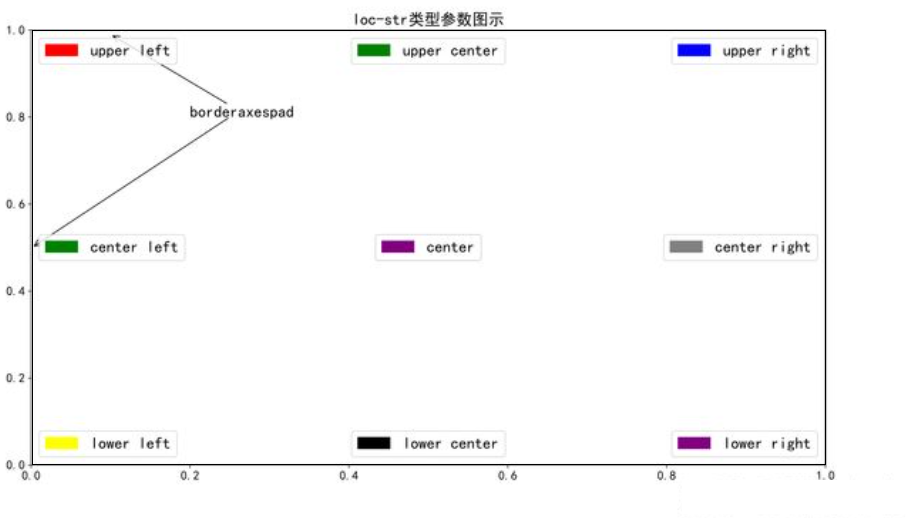例如将上例中,设置如下: 图例就显示在正中心

plt.legend(loc='center')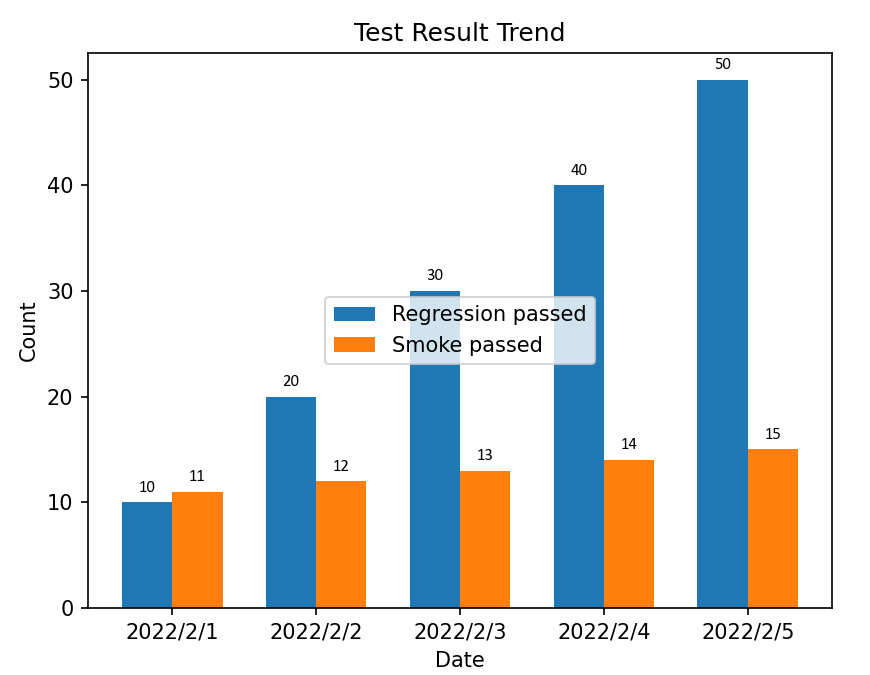bbox_to_anchor=(x0, y0) 自定义图例的起始坐标位置, 要结合 loc 这个参数使用, 它所处的方向就有 loc 这个参数来提供.

首先将 X, Y 轴看成是 (0,0) -> (1,1), 即 X, Y 轴看成长度分别是 1. 那么bbox_to_anchor(0.5, 0.5) 就是坐标轴中心的点.

还是拿上面的例子为例:

首先legend是一个bbox类型，是一个由4条边框围成的区域，轴域（axes)也是由4条边框围成的区域（x, y 轴，上边缘线，右边缘线）

plt.legend(bbox_to_anchor=(0.5, 1), loc='center')


坐标点 (0.5, 1), 它的方向是正中心,就是红点那个位置.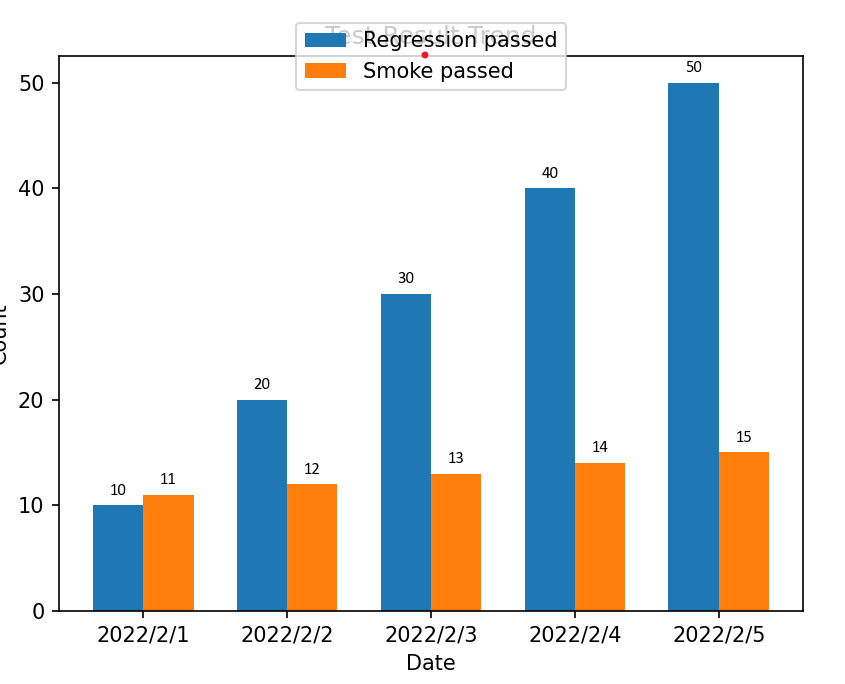改变一下方向: 作上角,坐标点(0.5, 1) 的点位于 图例矩形的左上角.

plt.legend(bbox_to_anchor=(0.5, 1), loc='upper left')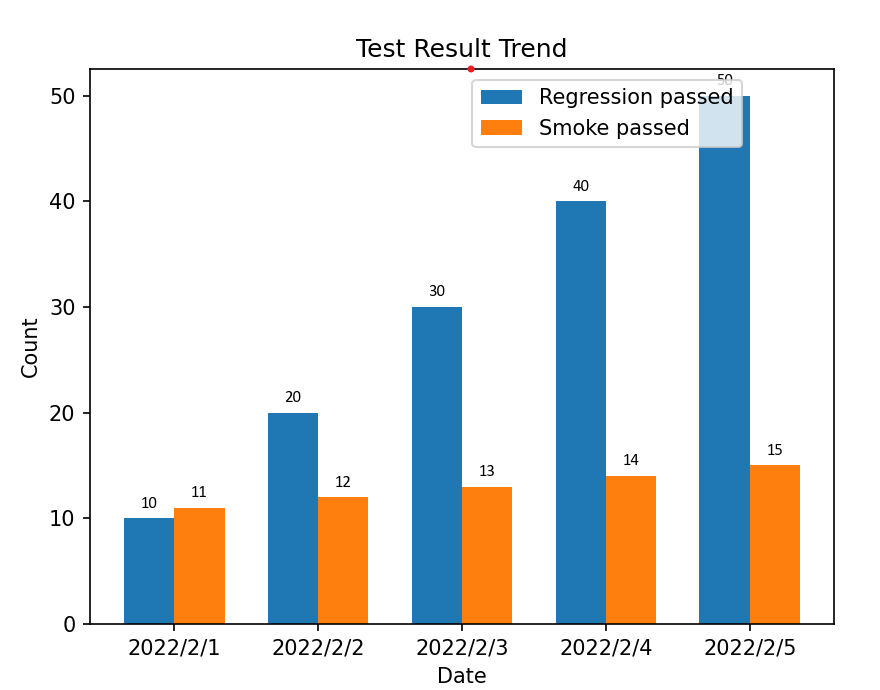改变左边轴和方向, 坐标点(1, 0.5) 就位于图例矩形左边中心位置.由于画板默认大小显示不全,你也可以通过 figure设置画板大小.

plt.legend(bbox_to_anchor=(1, 0.5), loc='center left')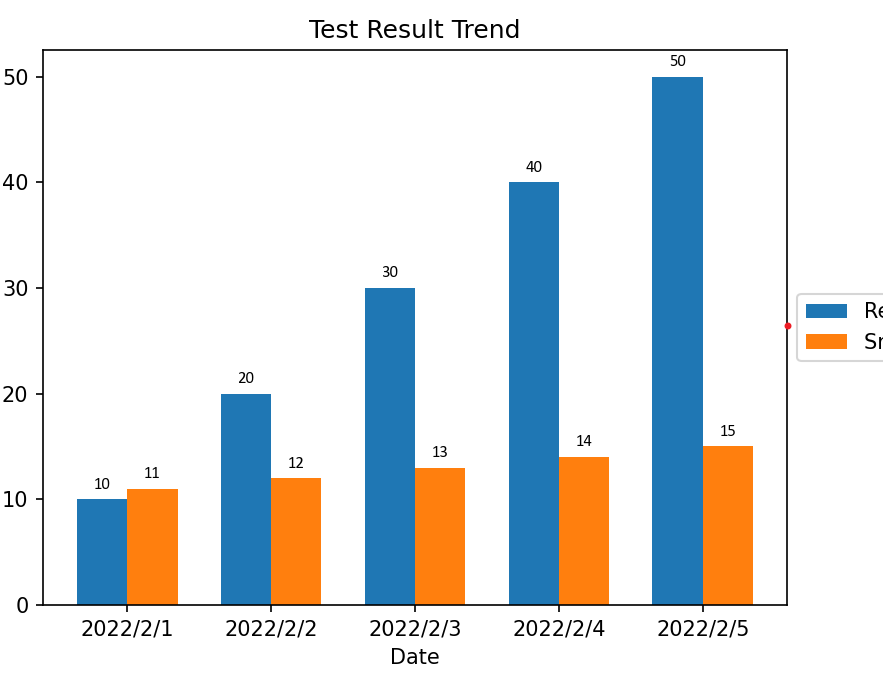# plt.gca() 坐标轴的设置

只画一个坐标轴，没有数据填充。

import matplotlib.pyplot as plt
plt.figure(figsize = (5,5))
plt.plot()
plt.show()


生成的图看起来有点不数据，因为不是标准的过原点 (0, 0)的坐标轴。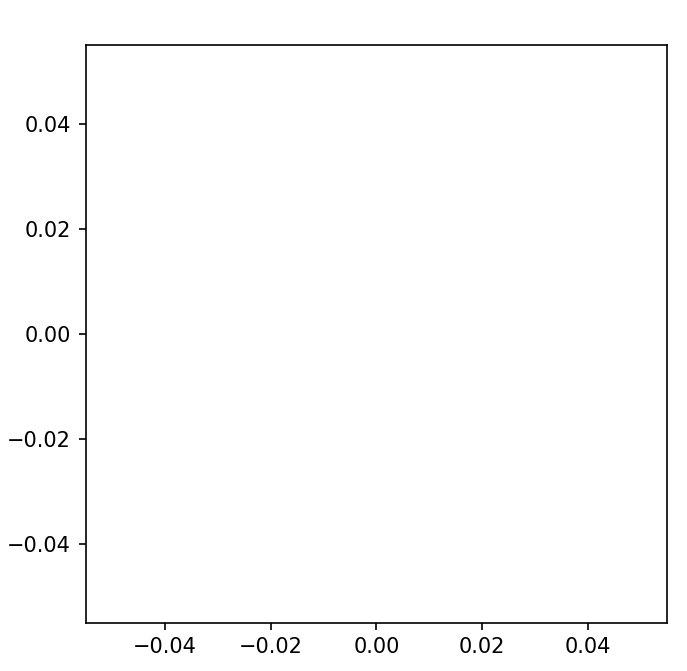gca 就是 get current axes 获取当前坐标轴，坐标轴是由左 Left，右 right，顶 top，底 bottom 4 个方向组成的。X 轴就是底部 bottom 的那根线，Y 轴就是左边 Left 的那根线。要移动某跟轴，需要先锁定某个方向。

例如，将 X 轴平移到 y=0 的位置上

import matplotlib.pyplot as plt
plt.figure(figsize = (5,5))
plt.plot()
ax = plt.gca()
#  要挪动底部的X轴，所以先目光锁定底部！
ax.xaxis.set_ticks_position('bottom')
# 'data'表示按数值挪动，其后数字代表挪动到Y轴的刻度值
ax.spines['bottom'].set_position(('data',0))
plt.show()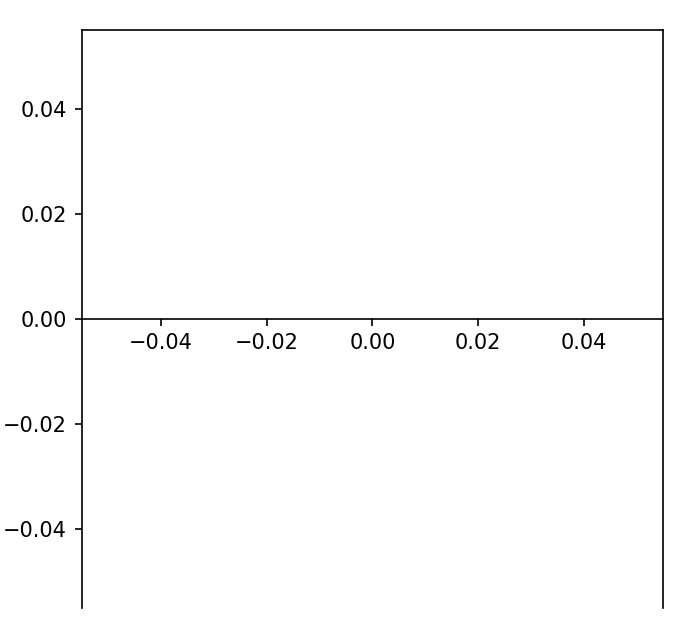同理移动 Y 轴

import matplotlib.pyplot as plt
plt.figure(figsize = (5,5))
plt.plot()
ax = plt.gca()
ax.yaxis.set_ticks_position('left')
ax.spines['left'].set_position(('data',0))
plt.show()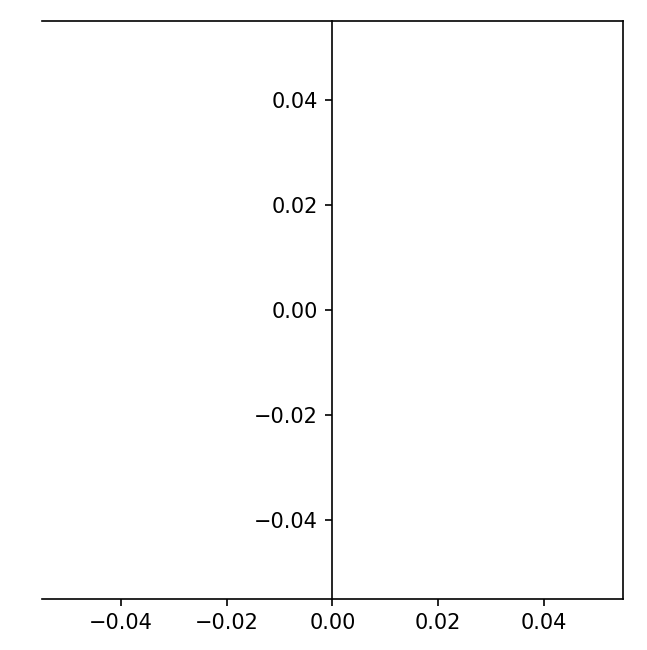也可以设置坐标轴在整个画板的比例，通过设置宽度和高度的比例，特别是当设置图例在画板两测是，图例可能显示不完整，所以适当的缩放坐标轴的占比

如原图，坐标轴在画板中的占比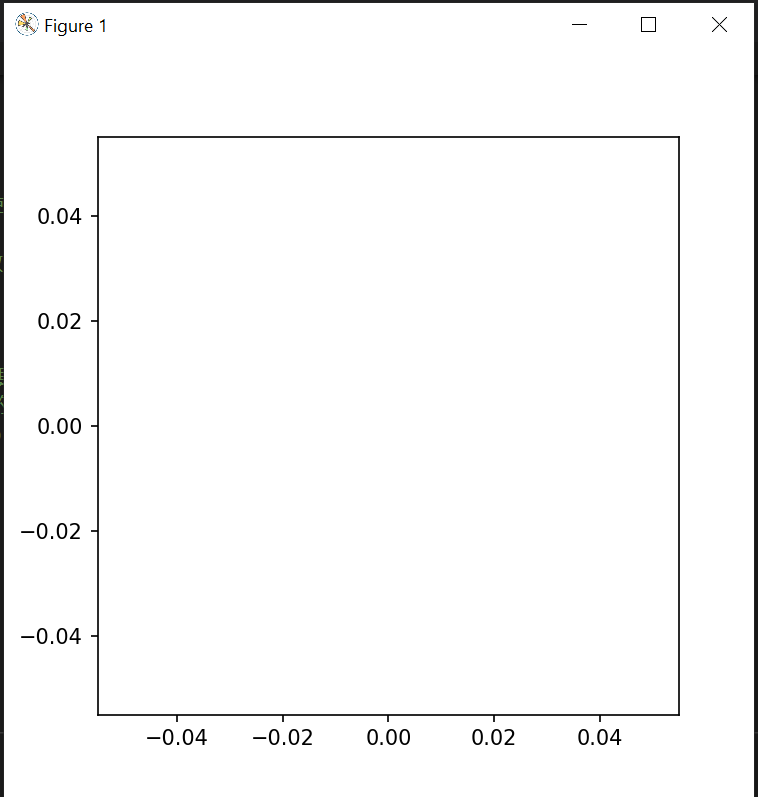将宽度设置成只占 90%

import matplotlib.pyplot as plt
plt.figure(figsize = (5,5))
plt.plot()
ax = plt.gca()
box = ax.get_position()
ax.set_position([box.x0, box.y0, box.width * 0.9, box.height])
plt.show()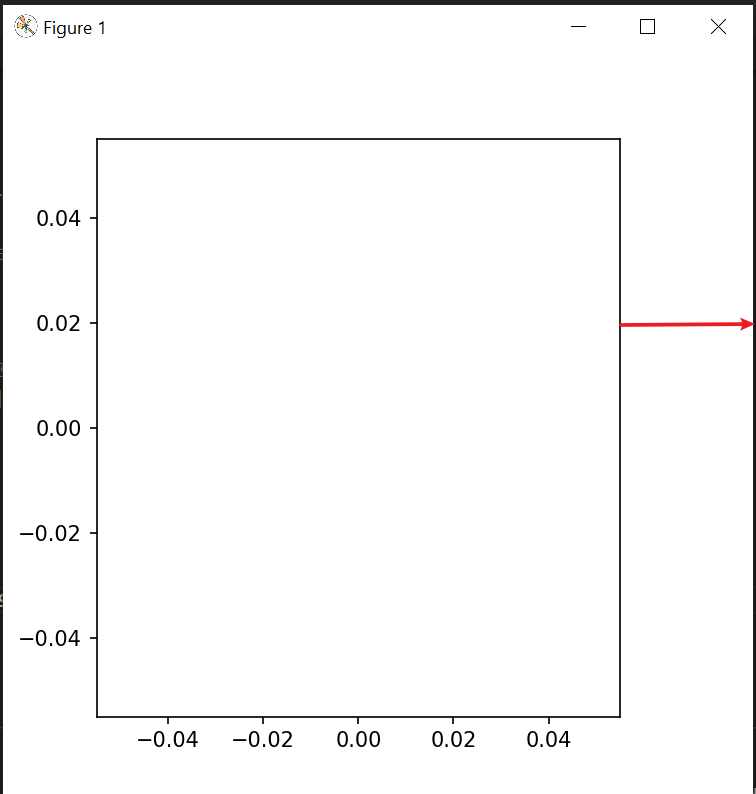# 一个完整的直方图例子

结合上面的知识点,来实践一下. 统计一下最近10天时间 regression 和 smoke 测试用例的运行趋势.
需求:
fail 的数据分别显示在 pass 的上方法,并用红色显示
图例位于画板右侧中部位置
直方图加上数据标签

先看一下效果图:
颜色灵感来自第 24 界北京冬奥会开幕式《立春》的绿色，哈哈！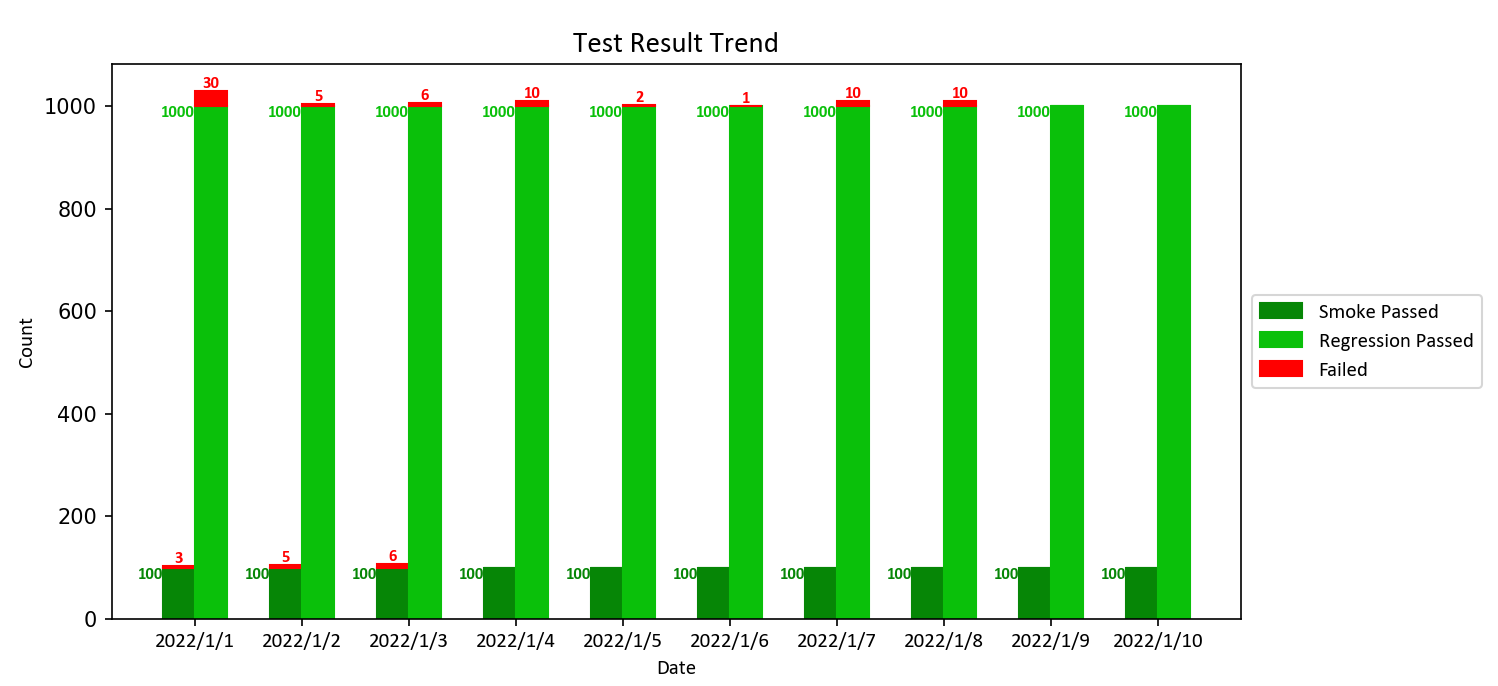## 小窍门：

如果数据悬殊比较大，比如大的数字非常大，小的数字非常小，在直方图上就显示不明显，绘制直方图的时候用巧妙用 edgecolor 这个属性。

plt.bar(x - bar_width/2, smoke_total_count_fail, color="red", edgecolor=smoke_fail_color, width= bar_width, bottom=smoke_total_count_pass)


当要保存图片时，需要注释掉 plt.show()，不然保存的图片会是空白。

plt.show() # should comment it if save the pic as a file, or the saved pic is blank
# dpi: image resolution, better resolution, bigger image size
# plt.savefig(image_file_name, bbox_inches='tight', dpi = 600)


## 完整代码

import matplotlib.pyplot as plt
import matplotlib.font_manager as font_manager
import numpy as np

def draw_trend_image(reg_total_count_pass, reg_total_count_fail, smoke_total_count_pass, smoke_total_count_fail, date_list, image_file_name = 'test_result_trend.png'):

# attributes
bar_width = 0.3
regression_pass_color = '#0ac00a'
smoke_pass_color = '#068606'
font_name = 'Calibri'
label_size = 10
text_size = 8
title_size = 14

# set the color for failure
smoke_fail_color = []
for item in smoke_total_count_fail:
if item > 0:
smoke_fail_color.append("red")
else:
smoke_fail_color.append(smoke_pass_color)

reg_fail_color = []
for item in reg_total_count_fail:
if item > 0:
reg_fail_color.append("red")
else:
reg_fail_color.append(regression_pass_color)

if len(date_list) == 10:
plt.figure(figsize=(10.8, 4.8))

# draw bar
x = np.arange(len(date_list))
plt.bar(x - bar_width/2, smoke_total_count_pass, color=smoke_pass_color, edgecolor=smoke_pass_color, width= bar_width, label="Smoke Passed")
plt.bar(x - bar_width/2, smoke_total_count_fail, color="red", edgecolor=smoke_fail_color, width= bar_width, bottom=smoke_total_count_pass)
plt.bar(x + bar_width/2, reg_total_count_pass, color=regression_pass_color, edgecolor=regression_pass_color, width= bar_width, label="Regression Passed")
plt.bar(x + bar_width/2, reg_total_count_fail, color="red", edgecolor=reg_fail_color, width= bar_width, label="Failed", bottom=reg_total_count_pass)

# set title, labels
plt.title("Test Result Trend", fontsize=title_size, fontname=font_name)
plt.xlabel("Date",fontsize=label_size, fontname=font_name)
plt.ylabel("Count",fontsize=label_size, fontname=font_name)
plt.xticks(x, date_list,fontsize=label_size, fontname=font_name)

# get current axes and set width ratio
ax = plt.gca()
box = ax.get_position()
ax.set_position([box.x0, box.y0, box.width * 0.9, box.height])

# loc: the position of the legend, bbox_to_anchor: start position of the legend
legend_font = font_manager.FontProperties(family=font_name, weight='normal',style='normal', size=label_size)
plt.legend(loc='center left', bbox_to_anchor=(1, 0.5), prop= legend_font)

# set bar text
for i in x:
if smoke_total_count_fail[i] > 0:
plt.text(i-bar_width/2, smoke_total_count_fail[i] + smoke_total_count_pass[i], smoke_total_count_fail[i],horizontalalignment = 'center', verticalalignment='bottom',fontsize=text_size,family=font_name,color='red',weight='bold')

plt.text(i-bar_width, smoke_total_count_pass[i], smoke_total_count_pass[i],horizontalalignment = 'right', verticalalignment='top',fontsize=text_size,family=font_name,color=smoke_pass_color,weight='bold')
plt.text(i, reg_total_count_pass[i], reg_total_count_pass[i], horizontalalignment = 'right', verticalalignment='top',fontsize=text_size,family=font_name,color=regression_pass_color,weight='bold')

if reg_total_count_fail[i] > 0:
plt.text(i+ bar_width/2, reg_total_count_fail[i] + reg_total_count_pass[i], reg_total_count_fail[i],horizontalalignment = 'center', verticalalignment='bottom',fontsize=text_size,family=font_name,color='red',weight='bold')

plt.show() # should comment it if save the pic as a file, or the saved pic is blank
# dpi: image resolution, better resolution, bigger image size
# plt.savefig(image_file_name, bbox_inches='tight', dpi = 600)

if  __name__ == '__main__':
#    reg_total_count_pass = [11641, 11641, 11641,11641,11641,11641,11641,11641,11641,11641]
reg_total_count_pass = [1000, 1000, 1000,1000,1000,1000,1000,1000,1000,1000]
reg_total_count_fail = [30, 5, 6,10,2,1,10,10,0,0]
#    smoke_total_count_pass = [963, 963, 963,963, 963, 963,963, 963, 963,963]
smoke_total_count_pass = [100, 100, 100,100, 100, 100,100, 100, 100,100]
smoke_total_count_fail = [3, 5, 6,0,0,0,0,0,0,0]
date_list = ['2022/1/1', '2022/1/2', '2022/1/3','2022/1/4','2022/1/5', '2022/1/6', '2022/1/7','2022/1/8', '2022/1/9', '2022/1/10']
draw_trend_image(reg_total_count_pass, reg_total_count_fail, smoke_total_count_pass, smoke_total_count_fail, date_list)

展开全文• 计算频数：给定一个序列t：hist = {}for x in t:...将其除以n即可把频数转换成频率，这称为归一化：n = float(len(t))pmf = {}for x, freq in hist.items():pmf[x] = freq/n绘制直方图：Vals, freqs = hist.Render...
• 掷骰子10000次，将数值的频率绘制直方图 die.py from random import randint class Die(): """骰子类""" def __init__(self,num_sides=6): """骰子默认为６面""" self.num_sides = num_sides; def ...
• 主要介绍了opencv python统计及绘制直方图的方法，小编觉得挺不错的，现在分享给大家，也给大家做个参考。一起跟随小编过来看看吧
• 主要介绍了python plotly绘制直方图实例详解,文中通过示例代码介绍的非常详细，对大家的学习或者工作具有一定的参考学习价值,需要的朋友可以参考下
• 1.在了解绘制直方图函数的参数之前，首先要知道如何绘制直方图： 一般而言，直方图一般用于观察数据的分布情况，横轴将根据观测到的数据选择合适的范围进行范围分段，即将整个观测数据的范围分成一系列间隔，每一个...
• 本篇文章给大家带来的内容是关于Python绘制直方图及子图的方法分析（代码示例），有一定的参考价值，有需要的朋友可以参考一下，希望对你有所帮助。1、直方图的绘制也需要用到matplotlib下的pylab，只不过在绘制折线...
• Python 绘制直方图 plt.hist(h) plt.show() rNumpy print('axis sum',rNumpy.sum(axis=1))#每个客户一共用的代数 h = rNumpy.sum(axis=1) num_bins = 300#一共135个人 plt.hist(h,num_bins, facecolor='...
• 1.基本概念1.1 直方图在统计学中，直方图（英语：Histogram）是一种对数据分布情况的图形表示，是一种二维統計圖表，它的两个坐标分别是统计样本和该样本对应的某个属性的度量。如果还是太抽象了，我们在来举一个...
• ## 用python绘制直方图

千次阅读 2020-11-20 19:36:29
其实，学习数据分析，excel可以完成绝大多数的工作，只是课程中@tiger是用python做的，而且最近自己也在学习编程，所以就挑战一下新东西，学学用python做作业，感谢@余欣 的教程，让我一个python小白也能上手做作业...
• 1.成绩可视化，输入成绩，绘制直方图 lis_score=[] n=int(input("输入学生人数：")) while n<1: print("学生人数需大于1") n = int(input("输入学生人数：")) def check_score(score): if score >= 0 and ...
• 这篇文章总的讲述了一下绘制频数直方图和频率直方图绘制方法，通过图像进行比较，发现各个直方图的优缺点，以便在工程中进行运用。matplotlib
• 其实直方图就是用来展现连续性数据分布特征的统计图形，通过绘制直方图，就可以很直观的观测出数据集中的各组成数据的特征、趋势和波动等情况哦。 在Python数据可视化中，直方图的绘制其实是通过调用 hist()函数来...编程语言 机器学习 人工智能
• matplotlib库作为Python的经典和最为常用的数据可视化库，它拥有强大的绘制图表的能力，最近...其实直方图就是用来展现连续性数据分布特征的统计图形，通过绘制直方图，就可以很直观的观测出数据集中的各组成数据的...
• 主要介绍了用Pygal绘制直方图代码示例，具有一定借鉴价值，需要的朋友可以了解下。
• 其实，学习数据分析，excel可以完成绝大多数的工作，只是课程中@tiger是用python做的，而且最近自己也在学习编程，所以就挑战一下新东西，学学用python做作业，感谢@余欣 的教程，让我一个python小白也能上手做作业......